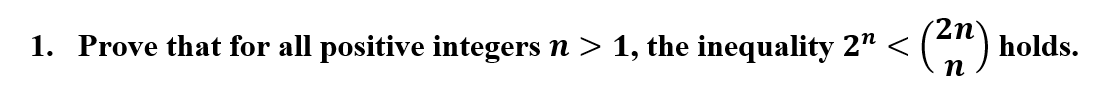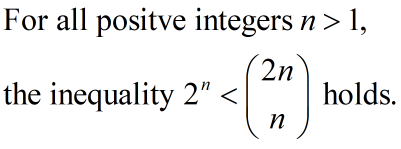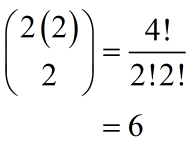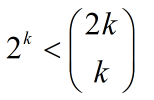# 2n°1. Prove that for all positive integers n > 1, the inequality 2" <

Question
1 viewshelp_outlineImage Transcriptionclose2n° 1. Prove that for all positive integers n > 1, the inequality 2" < <(") holds. п fullscreen
check_circle

Step 1

Consider the given statement be P(n) as;Apply induction methods to prove the above statement P(n) as follows.

For n=2,

LHS=22=4

RHSHere, 2<6. The statement is true for n=2.

Assume that the given statement is true for some natural number k.

That is,...

### Want to see the full answer?

See Solution

#### Want to see this answer and more?

Solutions are written by subject experts who are available 24/7. Questions are typically answered within 1 hour.*

See Solution
*Response times may vary by subject and question.
Tagged in

### Math